# ISEE Upper Level Quantitative : How to find the length of the diagonal of a rectangle

## Example Questions

### Example Question #1 : How To Find The Length Of The Diagonal Of A Rectangle

Which is the greater quantity?

(a) The length of a diagonal of a square with sidelength 20 inches

(b) The length of a diagonal of a rectangle with length 25 inches and width less than 10 inches

(a) is greater

(a) and (b) are equal

It is impossible to tell which is greater from the information given

(b) is greater

(a) is greater

Explanation:

The lengths of the diagonals of these rectangles can be computed using the Pythagorean Theorem:

(a)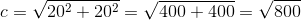(b)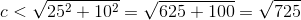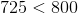so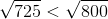. Since the diagonal of the rectangle in (b) measures less than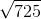, it must also measure less than that of the square in (a)

### Example Question #2 : How To Find The Length Of The Diagonal Of A Rectangle

In Rectangle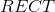,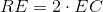, the diagonals intersect at a point.

Which is the greater quantity?

(a)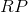(b)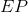(b) is greater.

(a) and (b) are equal.

(a) is greater.

It is impossible to tell from the information given.

(a) and (b) are equal.

Explanation:

The diagonals of a rectangle are congruent and bisect each other. Therefore,is equidistant from all four vertices, making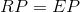. The relationship between the sides is not relevant here.

### Example Question #3 : How To Find The Length Of The Diagonal Of A Rectangle

Rectanglehas length 60 inches and width 80 inches. The two diagonals of the rectangle intersect at point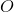. Which is the greater quantity?

(a)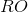(b)(b) is greater.

(a) is greater.

It is impossible to tell from the information given.

(a) and (b) are equal.

(a) is greater.

Explanation:

Two consecutive sides of a rectangle and a diagonal form a right triangle, so the length of any diagonal can be determined using the Pythagorean Theorem, substituting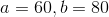: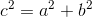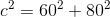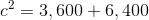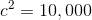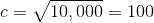The diagonals of a rectangle bisect each other. Therefore, the distance from a vertex to the point of intersection is half this, and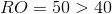.

### Example Question #4 : How To Find The Length Of The Diagonal Of A Rectangle

A rectangle has perimeter 140 inches and area 1,200 square inches. Which is the greater quantity?

(A) The length of a diagonal of the rectangle.

(B) 4 feet

It is impossible to determine which is greater from the information given

(A) and (B) are equal

(A) is greater

(B) is greater

(A) is greater

Explanation:

Letand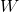be the dimensions of the rectangle. Then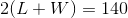and, subsequently,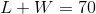Since the product of the length and width is the area, we are looking for two numbers whose sum is 70 and whose product is 1,200; through trial and error, they are found to be 30 and 40. We can assign either to beand the other to besince the result is the same.

The length of a diagonal of the rectanglecan be found by applying the Pythagorean Theorem: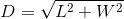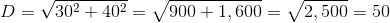A diagonal is 50 inches long; since 4 feet are equivalent to 48 inches, (A) is the greater quantity.